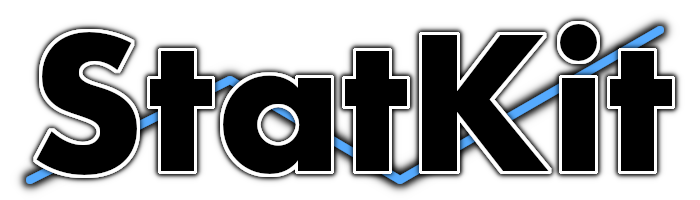# Swiftpack.co - JimmyMAndersson/StatKit as Swift Package

Swiftpack.co is a collection of thousands of indexed Swift packages. Search packages.
A collection of statistical analysis tools for your Swift programs.
⭐️ 39
🕓 1 week ago
iOS macOS tvOSWelcome to StatKit, a collection of statistical analysis tools for Swift developers. StatKit adds tools for statistical analysis directly to the Swift types you use every day, such as Array, Set, and Dictionary.

## Builtin Statistics

StatKit extends the Swift standard library to include relevant functionality for statistical analysis in the types you work with every day. Using StatKit, you will be able to calculate a variety of useful statistics such as:

• Central Tendency
Calculate modes, means, and medians of your data collections.

• Variability
Compute variances and standard deviations.

• Correlation
Find linear tendencies and covariance of measurements.

• Distributions
Make computations using several common distribution types.

A simple example would be to calculate the modes of an integer array, which can be done easily with the following piece of code:

``````print([1, 2, 3, 3, 2, 4].mode(of: \.self))

// Prints [3, 2]
``````

In this case, `mode(of:)` takes a KeyPath argument which specifies the variable inside the array that you are interested in. In the example above, we specify the `\.self` keypath, which points to the array element itself (in this case, the integers).

The pattern of specifying one or more variables to investigate is common throughout the StatKit library. It allows you to calculate similar statistics for a variety of different types using the same syntax. For example, both of the below examples produce valid results, even though the types under investigation are completely disparate:

Calculating the mode of all characters in a `String`:

``````print("StatKit".mode(of: \.self))

// Prints ["t"]
``````

Calculating the mode of `CGPoint` y-values in an array:

``````import CoreGraphics

let points = [CGPoint(x: 0, y: 1),
CGPoint(x: 1, y: 3),
CGPoint(x: 3, y: 1)]

print(points.mode(of: \.y))
// Prints [1.0]
``````

## Computing statistics for collections of complex custom types

As the examples in the previous section showed, calculating statistics is easy when using collections of types that are readily available. However, most of us work with custom data structures in our projects. Luckily, StatKit provides support for arbitrary custom types thanks to the extensive use of generics.

Let us look at a custom data structure that keeps track of collected data points for a specific brand of cars, and how we can use StatKit to wasily calculate the mean and standard deviation of their fuel consumption:

``````struct FuelConsumption {
let modelYear: String
let litersPer10Km: Double
}

let measurements: [FuelConsumption] = [...]

measurements.mean(.arithmetic, of: \.litersPer10Km)
measurements.standardDeviation(of: \.litersPer10Km, from: .sample)
``````

As you can see, using KeyPath's makes the StatKit API easy to use and reusable across completely arbitrary custom structures.

## Distributions

StatKit provides multiple discrete and continuous distribution types for you to work with. These allow you to compute probabilities, calculate common moments such as the skewness and kurtosis, and sample random numbers from a specific data distribution.

``````let normal = NormalDistribution(mean: 0, variance: 1)
print(normal.cdf(x: 0))
// Prints 0.5

let normalRandomVariables = normal.sample(10)
// Generates 10 samples from the normal distribution
``````

## System Requirements

To use StatKit, make sure that your system has Swift 5.5 (or later) installed. If you’re using a Mac, also make sure that `xcode-select` points at an Xcode installation that includes a valid version of Swift and that you’re running macOS Catalina (10.15) or later.

IMPORTANT
StatKit does not officially support any beta software, including beta versions of Xcode and macOS, or unreleased versions of Swift.

## Installation

### Swift Package Manager

To install StatKit using the Swift Package Manager, add it as a dependency in your `Package.swift` file:

``````let package = Package(
...
dependencies: [
],
...
)
``````

Then import StatKit where you would like to use it:

``````import StatKit
``````

### Cocoapods

To install StatKit using Cocoapods, simply add `pod 'StatKit'` to your Podfile. To use a specific version, check out the Podfile reference for the appropriate syntax.

``````target 'MyApp' do
use_frameworks!
pod 'StatKit'
end
``````

## Contributions and support

StatKit is a young project that is under active development. Our vision is to create the go-to statistics library for Swift developers, much like SciPy and NumPy are for the Python language.

:heart: Consider becoming a sponsor to support the development of this library.
You could cover an afternoon coffee or a meal to keep my neurons firing.

Thank you for your contribution, and enjoy using StatKit!

### GitHub

 link Stars: 39 Last commit: 1 week agoSomething's broken? Yell at me @ptrpavlik. Praise and feedback (and money) is also welcome.

### Release Notes

StatKit beta 4
14 weeks ago

Welcome to the StatKit beta. StatKit beta 4 brings new functionality to the standard library components you use every day.

New in this release:

• The error of computations is now less than 1e-6.
• Gamma and Beta functions.
• Discrete uniform distribution.
• Geometric distribution.
• Binomial distribution and coefficient.
• Up to 10x performance improvement when generating random samples!

Bug fixes:

• Poisson distributions now include x = 0.

Feel free to help me develop and maintain this library if you like it! https://github.com/sponsors/JimmyMAndersson

Thank you, and enjoy using StatKit!

Swiftpack is being maintained by Petr Pavlik | @ptrpavlik | @swiftpackco | API | Analytics